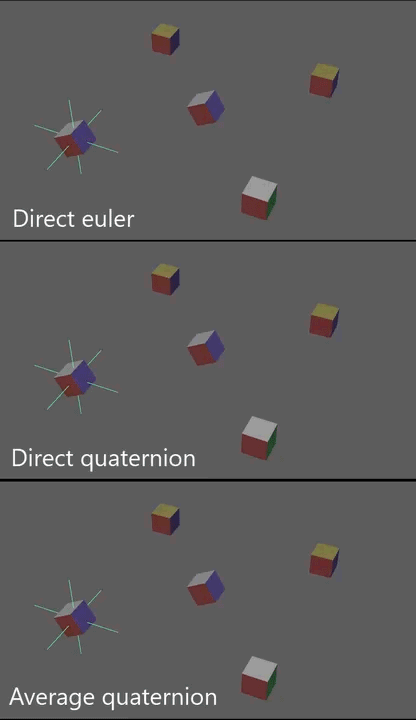# Quaternion Rotation Output from RBF Solvers

In my previous post, I described how driving an RBF solver with quaternion values for rotational inputs is more reliable than driving with euler inputs. In this post, I will describe a method to output rotational values from an RBF solver.

As described in my last post, euler rotations suffer from gimbal lock and multiple solutions to the same rotation. If we were to solve directly to these euler angles, we would see a lot of undesirable flipping depending on the sample inputs.

We can try solving to quaternion components directly, however quaternions also suffer from having multiple solutions (two) for the same rotation.

A solution is to use quaternion averaging.You may have heard that since quaternion multiplication is not commutative, it is impossible to combine multiple rotations in an order-independent way. While this is true when multiplying quaternions together, there is a way to calculate the weighted average of multiple quaternions described in the paper, Averaging Quaternions, written by really smart aerospace engineers and research professors.

Basically, given a 4xN matrix where each column is the scaled quaternion:

$$Q = \begin{bmatrix} a_{1}q_{1} & a_{2}q_{2} & a_{3}q_{3} & \cdots & a_{n}q_{n} \end{bmatrix}$$

The weighted average quaternion is the normalized eigenvector corresponding to the largest eigenvalue of

$$QQ^T$$

VectorXd averageQuaternion(const MatrixXd& inputQuats, const VectorXd& weights) {
MatrixXd Q = inputQuats * weights;
Q *= Q.transpose();
auto eigenValues = solver.eigenvalues();
double maxValue = eigenValues;
int maxIndex = 0;
for (int j = 0; j < eigenValues.size(); ++j) {
if (eigenValues[j] > maxValue) {
maxValue = eigenValues[j];
maxIndex = j;
}
}
VectorXd averageQuat = solver.eigenvectors().col(maxIndex);
return averageQuat;
}


Note that the weights should be normalized and add up to 1. Also, don't worry if you don't fully understand what eigenvalues and eigenvectors are. I know I haven't used them much since my college linear algebra and statistics classes so I am no expert either.

To support solving for the average quaternion, we need to make some adjustments to the RBF solver described in the last couple of posts. Previously we solved for the output values directly. What we want to do instead is solve for a weight value for each sample. For example if we have 3 samples, we will use output values of

$$\begin{bmatrix} 1 & 0 & 0 \\ 0 & 1 & 0 \\ 0 & 0 & 1 \end{bmatrix}$$

This ends up being an identity matrix the size of the number of samples. The first row means, when we are exactly on the first sample, the first sample should have a weight of 1 and the second and third samples should have weight 0.

When calculating the theta coefficients in the linear regression equation, we use an identity matrix instead of the sample output values.

// Rather than solve directly to the output values, we will store 0 or 1 pose values.
// This lets us calculate the output as a linear combination of the sample outputs and
// will make it easier to calculate output quaternions
MatrixXd outputMatrix = MatrixXd::Identity(sampleCount, sampleCount);
MatrixXd r = MatrixXd::Zero(cols, cols);
r.diagonal().array() = regularization;

MatrixXd tm = m.transpose();
MatrixXd mat = pseudoInverse(tm * m + r) * tm;
theta_ = (mat * outputMatrix).transpose();


Once we have solved the system as described in previous posts, we can calculate the actual output values for both scalar output and rotational output using the calculated sample weights. Scalar output is just a linear combination of the calculated sample weights and the actual output values:

VectorXd output = theta_ * inputDistance;
int outputCount = outputScalarMatrix_.cols();
outputs.resize(outputCount);
for (unsigned int i = 0; i < outputCount; ++i) {
outputs[i] = output.dot(outputScalarMatrix_.col(i));
}


To calculate the average quaternion, we make sure the sample weights are normalized and then we can calculate the average quaternion using the function described above.

int outputQuatCount = outputQuats_.size();
output.normalize();  // Weights must be normalize for weight avg of quaternions
outputQuats.resize(4, outputQuatCount);
for (unsigned int i = 0; i < outputQuatCount; ++i) {
outputQuats.col(i) = averageQuaternion(outputQuats_[i], output);
}


Using the average quaternion gives us a more stable interpolation when outputting rotation from the RBF solver as seen in the image in this post. To view the full code listing, refer to my GitHub